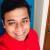true

Find the best tutors and institutes for Class 10 Tuition

Find Best Class 10 Tuition

No matching category found.

No matching Locality found.

Outside India?

Search for topics

# Number SystemSuvam Banerjee
06 Mar 00

Natural Numbers: The set of counting numbers is called natural numbers. It is denoted by N, where N = {1,2,3, …..}

Whole Numbers: when zero is included in the set of natural numbers, then it is a set of whole numbers. It is denoted by W, where W = {0,1,2, …..}

Integers: When in the set of whole numbers, natural numbers with the negative sign are included, then it becomes a set of integers. It is denoted by Z, where Z = {-…. , -2, -1, 0, 1, 2, …..}

Integers can be divided into negative and positive integers.

Prime Numbers: The natural numbers which have no factors other than 1 and itself are called prime numbers.

Co-prime Numbers: Two numbers that have no common factor except one are called co-prime numbers. Example: 11 and 18 etc.

Rational Numbers: The numbers that can be expressed in the form of p/q where p and q are integers and co-prime are called rational numbers. It is denoted by Q. Rationalnumbers can be positive or negative.

Irrational Numbers : The numbers which are not rational numbers, are called irrational numbers. or π = 3.141592 is an irrational number.

Real Numbers : Set of all rational numbers as well as irrational numbers are called real numbers. The square of all real numbers is positive.

Some important points on numbers :

• 2 is the only even prime number.
• Number 1 is neither divisible nor prime.
• Two consecutive odd prime numbers are called prime pair.
• All natural numbers are whole, rational, integer and real.
• Fractions are rational.
• 0 is neither negative(-) nor positive(+) number.
• Dividing any number by 0 gives infinity () whereas dividing 0 by any number gives 0.
• The sum and product of two rational numbers is always a rational number.
• The product and sum of a rational number and irrational number is always an irrational number.
• There can be infinite numer of rational and irrational numbers between two rational numbers and two irrational numbers.

0 Dislike
Follow 2## Other Lessons for You

Mastering Simple Calculations, my way!
Did you ever wonder how you can master simple calculations? 37 + 49? let's add 3 to 37 making it 40 and then subtracting 3 from 49 making it 46. Here it is 46 + 40 = 86 which is same as 37 + 49 = 86. ...How to study Mathematics and not be afraid of the fun field.
Math Phobia or math anxiety is a very real problem that many young students and even adult professionals face. What actually is Math Phobia? It is the doubt on one's mathematical abilities. How does...Goods and Service Tax
Goods and Services Tax (GST)It is an indirect tax levied by the Central Government. In this tax ·there are so many taxesmerged such as Excise Duty, Customs Duty, Service Tax, Value Added Tax,Entertainment...### Looking for Class 10 Tuition ?

Find best Class 10 Tuition in your locality on UrbanPro.

Are you a Tutor or Training Institute?

Join UrbanPro Today to find students near you
X

### Looking for Class 10 Tuition Classes?

Find best tutors for Class 10 Tuition Classes by posting a requirement.

• Post a learning requirement
• Get customized responses
• Compare and select the best### Looking for Class 10 Tuition Classes?

Find best Class 10 Tuition Classes in your locality on UrbanProUrbanPro.com is India's largest network of most trusted tutors and institutes. Over 55 lakh students rely on UrbanPro.com, to fulfill their learning requirements across 1,000+ categories. Using UrbanPro.com, parents, and students can compare multiple Tutors and Institutes and choose the one that best suits their requirements. More than 7.5 lakh verified Tutors and Institutes are helping millions of students every day and growing their tutoring business on UrbanPro.com. Whether you are looking for a tutor to learn mathematics, a German language trainer to brush up your German language skills or an institute to upgrade your IT skills, we have got the best selection of Tutors and Training Institutes for you. Read more